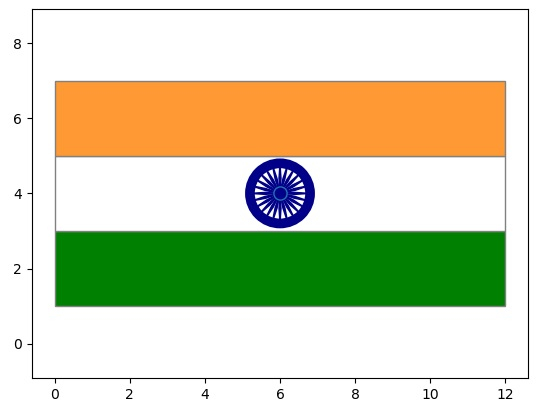Program to make Indian Flag in Python

PythonServer Side ProgrammingProgramming

Python’s libraries to draw graphs has very extensive features which can not only give us charts but also give us flexibility to draw other diagrams like flags. In that sense those modules have an artistic touch. In this article we will see how to draw the Indian flag using the libraries numpy and matplotlib.

Appraoch

• We create three rectangles of same width and draw them with appropriate colours and borders.

• Use pyplot function to draw the circle of the Ashok Chakra at the center of the middle rectangle.

• Use numpy and matplotlib to draw the 24 lines inside the Ashok Chakra.

• In all the above drawing we mention the colours, borders, radius and line lengths to match the requirement of the final size of the flag we need.

Program

We implement the above approach using the following program.

Example

import numpy as np
import matplotlib.pyplot as py
import matplotlib.patches as patch
#Plotting the tri colours in national flag
a = patch.Rectangle((0,1), width=12, height=2, facecolor='green', edgecolor='grey')
b = patch.Rectangle((0,3), width=12, height=2, facecolor='white', edgecolor='grey')
c = patch.Rectangle((0,5), width=12, height=2, facecolor='#FF9933', edgecolor='grey')
m,n = py.subplots()
#AshokChakra Circle
py.plot(6,4, marker = 'o', markerfacecolor = '#000088ff', markersize = 9.5)
chakra = py.Circle((6, 4), radius, color='#000088ff', fill=False, linewidth=7)
#24 spokes in AshokChakra
for i in range(0,24):
p = 6 + radius/2 * np.cos(np.pi*i/12 + np.pi/48)
q = 6 + radius/2 * np.cos(np.pi*i/12 - np.pi/48)
r = 4 + radius/2 * np.sin(np.pi*i/12 + np.pi/48)
s = 4 + radius/2 * np.sin(np.pi*i/12 - np.pi/48)
t = 6 + radius * np.cos(np.pi*i/12)
u = 4 + radius * np.sin(np.pi*i/12)
n.add_patch(patch.Polygon([[6,4], [p,r], [t,u],[q,s]], fill=True, closed=True, color='#000088ff'))
py.axis('equal')
py.show()

Output

Running the above code gives us the following result −